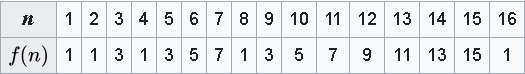# Josephus Problem in O(1) Time

## Problem Statement :

In computer science this is counting puzzle with count-out logic. It is similar to popular Netflix Drama, Squid Game. So the question goes like :

There will be n chairs, numbered 1 to n. Person sitting on the first chair will kill the next person(sitting on the second chair) and will hand over the sword to the person sitting on the third chair. This activity keeps on going in a loop, until there’s one survivor on some chair numbered say c. Your task to find that chair numbered c, where you can sit and survive this deadly game.

PROBLEM DRY RUN :

→ 1,2,3,4 and 5 are alive at initial state.

→ on the first pass, 1 kills 2, sword is passed to 3.

→ on the second pass, 3 kills 4 and sword is passed to 5.

→ on the third pass, 5 kills 1 and sword is given to 3.

→ in the last fourth pass, 3 kills 5 and 3 survives.

— -GAME OVER — -

## Next Step : To find out some pattern based upon problem series analysis :o/p for different values of n

## Pattern to note here :

→ For every number, which is a power of 2, the person not killed is the one from where the game begin, i.e chair 1. Example n = 1,2,4,8,16. Here f(n) =1

→ In the series, value of f(n) is odd number for the case where n is not a power of 2. The series keeps on increasing and comes back to 1, when n is a power of 2.

Initial intuition : If n is a power of 2, in that case, person who will survive will be person who starts the game.

Induction : if n = 2^k, then f(n)=1, if game starts with chair numbered 1.

Proof: Assume n = 8. Here in first go, all the even positioned person(even because the problem says, kill next, pass the sword to next’s next) will be killed, that are 2,4,6,8(Remaining 1,3,5,7). Sword comes back to 1 and again all the people who are left in game and are at even position(3,7) are killed and sword comes back to 1. Lastly 5 is killed by 1. Hence for n=2^k, if game starts with chair c, f(n)=c.

Next intuition : For all n, we can express n = 2^k +p. So if we kill p game members, crossing 2p chairs(even positioned), then the chair 2p+1 will be f(n) as it will be the chair number from where rest of the 2^k members begin. And as per : for n=2^k, if game starts with chair c, f(n)=c.

Example : if n = 15, it can be expressed as 2³ + 7. So we have to kill 7 people start the 2³ series. Which mean, we have to cross 2*7=14 chairs to kill 7. So the person who will survive will sit on 2*7 +1 = 15th chair, f(n)=15

Hence from the above proof, we can take as chair numbered c = f(n) = 2*p+1, where n = 2^K +p. This formula can be used to solve the problem in constant time.

Hope you had an amazing problem solving round :) Many more stories ahead, so keep following!

Follow me on medium and LinkedIn(linkedin.com/in/shiwani-sinha-28b842171), to get more interview preparation materials and insights.

Thanks!

--

--## Shiwani Sinha 🍁

💻| Software Engineer @microsoft 🧋| Fuelled by Caffeine ❤️| I thought you'd like the ideas I've been saving on Pinterest... ⬇️ https://pin.it/3oP8voW